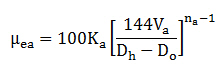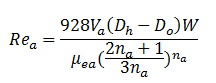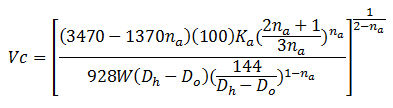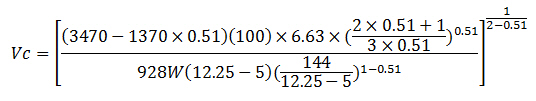# Critical Flow Rate – Drilling Hydraulics

Critical flow rate is the flow rate at the transition point between laminar and turbulent flow. The first step of the critical flow rate determination is to figure out the critical velocity and then substitute it into the annular flow rate.

To get the point at the transition period, the critical Reynold Number for laminar flow must be around 3470 – 1370na. With this relationship, we can determine the critical velocity by rearranging the Reynold Number and Effective Viscosity equation.

The effective viscosity equation for critical velocity is listed below:The Reynolds number equation for critical velocity is listed below:The critical annular velocity equation is listed below:Where;

Vc = critical velocity in ft/sec

Rec = Reynold Number in the annulus

µea = effective viscosity in the annulus, centi-poise

na = power law constant (flow behavior index)

Ka = power law constant (consistency factor)

W = mud weight, ppg

Dh = Diameter of wellbore, inch

Do = Outside Diameter of tubular, inch

After we get the critical velocity, we can figure out the critical flow rate by the following equation.Where;

Qc = Critical flow rate, gpm

Vc = critical velocity in ft/sec

Dh = Diameter of wellbore, inch

Do = Outside Diameter of tubular, inch

Please follow the step-by-step calculation to learn how to determine the critical flow rate.

Power law constant (flow behavior index), na = 0.51

Power law constant (consistency factor), Ka = 6.63

Mud weight = 10 ppg

Hole diameter = 12-1/4”

Drill pipe size = 5”

Determine the critical annular velocity by substituting factors into this equation.Vc = 3.82 ft/sec

Then we can figure out the critical flow rate.Qc = 1172 GPM

Reference:Drilling Hydraulic Books

Share the joy